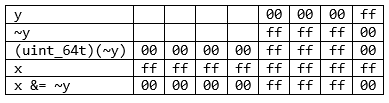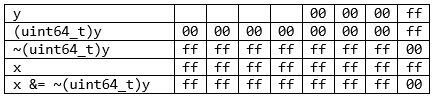﻿ V784. The size of the bit mask is less than the size of the first operand. This will cause the loss of the higher bits.# V784. The size of the bit mask is less than the size of the first operand. This will cause the loss of the higher bits.

The analyzer detected a suspicious operation performed on a bit mask: the bit mask is represented by a variable whose size is less than that of the other operand. This guarantees the loss of the value of high-order bits.

Consider a few examples that trigger this warning:

``````unsigned long long x;
unsigned y;
....
x &= ~y;``````

Let’s see in detail what happens to the bits after each operation using the following expression as an example:

``````x = 0xffff'ffff'ffff'ffff;
y = 0xff;
x &= ~y;``````A result like that is usually different from what the programmer expected:

``````0xffff’ffff’ffff’ff00 – expected result
0x0000’0000’ffff’ff00 – actual result``````

The code can be fixed by explicitly casting the 'y' variable to the type of the 'x' variable:

``x &= ~(unsigned long long)y;``

In this case, the type conversion will be executed first, followed by the negation. After that, all the most significant bits will be set to one. The following table shows how the result of the code above will change with the new order of computations:The analyzer also outputs the warning for code like this:

``````unsigned long long x;
unsigned y;
....
x &= y;``````

Even though no additional operations are performed here, this code still looks suspicious. We recommend using explicit type conversion to make the code’s behavior clearer to both the analyzer and your colleagues.

 According to Common Weakness Enumeration, potential errors found by using this diagnostic are classified as CWE-197.
 You can look at examples of errors detected by the V784 diagnostic.

410
14 111

### Do you make errors in the code?